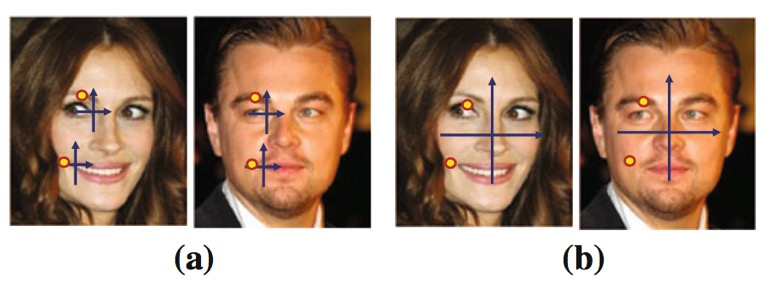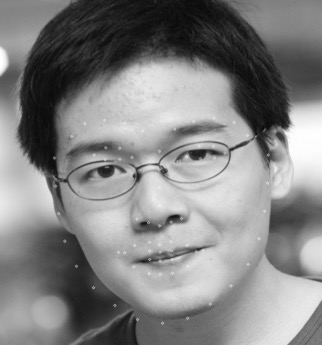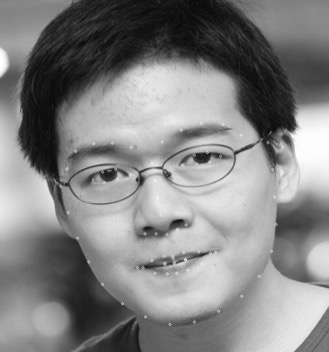C++实现和解读Face Alignment at 3000fps via Local Binary FeatureBinbin Xu 6月 07, 2015

C++实现代码的error和论文一致(最近又新添加了一个example，重写了一些代码)，详见我的github链接

## 1.Framework

1. 每个stage先抽取local binary features，
2. 然后根据真实的$\varDelta {\hat{S}}_i$ 用linear regression训练一个regressor，
3. 最后用训练出来的regressor得到$\varDelta S_i$(是$\varDelta {\hat{S}}_i$的近似)去更新前一个stage的shape，得到更加精确的shape

### 1.1 Training Phase:

Input: Image set {$I$}(N samples), ground truth shapes {$\hat{S}$}, initial shapes set {$S^0$}
For t=1:T do
$features_i = \phi^t (I_i,S_i^{t-1})$
$\varDelta \hat{S_i}=\hat{S_i} - S^{i-1}$
$E=\sum {\lVert \hat{S_i} - R^t(features_i)\rVert}^2$
$\varDelta S_i=R^t(features_i)$
$S^t_i=S^{t-1}_i+\varDelta S_i$
End For

### 1.2 Testing phase

Input: Image I, initial shape $S^0$
Output: refined shape $S$
For t=1:T do #总共有T个stage
$features = \phi^t(I,S^{t-1})$
$\varDelta S=R^t(features)$
$S^t=S^{t-1}+\varDelta S$
End For

## 2. 抽取Local Binary Features

1. 先random产生500个pixel difference features
2. 选取最具有分辨力的pixel difference features作为Random Forest中每棵树中的非叶子结点
3. 输入图片得到Local Binary Features

### 2.1 Pixel Difference Features

Pixel Difference Features源自CVPR 2012的一篇叫face alignment by explicity shape regression的文章。

1. Pixel Indexed Features如上图，作者认为对于特定的一个landmrk，它周围的有些点是几何不变的，比如我们有一个landmark是左眼的右眼角$P(x,y)$，在它的上方某个地方$\varDelta P(\varDelta x, \varDelta y)$具有不变的性质,比如附近的眉毛某点 $P(x+\varDelta x, y+\varDelta y)$ 就是黑色的,这个对每个人来说都是一样，当然由于光照等其他因素的原因，这个颜色还是有很大差异的，那么作者就提出了 Pixel Difference Features
2. Pixel Difference Features
其实很简单，就是将附近某两个点的值相减得到一个差值，而这个值很大程度上在一个阈值内浮动，而且还可以剔除光照等因素的影响，即
$$feature = I(x+\varDelta x_1, y+\varDelta y_1)-I(x+\varDelta x_2, y+\varDelta y_2)$$

### 2.2 创建Random Forest

Random Forest维基链接。Random Forest由很多tree组成，相比于单棵tree能够防止模型的over fitting。Random Forest能用于regression(本文用到的功能)和classification。

1. 首先我们确定一个landmark l，随机产生在l附近的500个pixel difference features的位置，然后对training中的所有images抽取这500个features，
2. 确定要构建l的第几个棵树（其他树一样，只是训练数据不一样而已）
3. 从树根节点开始
var = variance of landmark l of traing images,
var_red = -INFINITY, fea = -1, left_child = NULL, right_child = NULL
For each feature f:
threshold = random choose from all images’s feature f
// 比如现在所有图的f的值是2，2，2，4，5，3（假设有5张图），那么随机选择threshold可能是3
tmp_left_child = images with f < threshold
// 左子节点为所有f小于threshold的图片
tmp_right_child = images with f >= threshold
tmp_var_red = var - |left_child|/|root|*var_tmp_left_child - |right_child|/|root|*var_tmp_right_child
// var_tmp_left_child是左子节点landmark l的variance
if ( tmp_var_red > var_red) {
mvar_red = tmp_var_red
fea = f
left_child = tmp_left_child
right_child = tmp_right_child
}
End For
实际上var是固定的，所以不用算，|left_child|是当前left_child所包含的图片数，|root|表示root包含的图片数，实际计算的时候可以省去，因为是定的。
fea就是最后选择的feature
4. 对子节点left_child和right_child做跟3一样的操作，直到达到tree的最大depth，或者对于某一个根节点根据maximum variance reduction找到的feature是恰好一个child包含了所有的图，而另一个child没有图（事实上这个情况基本上不太可能出现），所以训练的时候基本上能够达到定义的max_depth，经我个人验证max_depth=5,6就可以了，在深很容易出现overfitting的问题
5. landmark l的其他树也一样如上，对其他landmark和对l的操作一样

### 2.3 Local Binary Features$$\phi^t = [\phi_1^t, \phi_2^t,…,\phi_L^t]$$

## 3. Learning Global Linear Regression

$\min_{W^t} {\sum_{i=1}^N} {\lVert \varDelta \hat{S_i^t} - W^t\phi^t(I_i,S_i^{t-1}) \rVert}^2_2+\lambda{\lVert W^t \rVert}^2_2$

1. 比如对于第一个landmark的$\varDelta x$坐标进行regression，输入就是所有图片的local binary feature矩阵，所有$\varDelta x$坐标组成的vector作为regression target，最后可以得到一个权重向量w，然后有了新的图片，抽取它的local binary feature后，乘以w就可以得到预测的$\varDelta x$值，最后加到上一个stage的$x$上面得到新的$x$
2. 对于第一个landmark的$\varDelta y$也是一样，这里的local binary feature矩阵和上面$\varDelta x$矩阵的一样，只是要regression的target $\varDelta y$不一样，
3. 其他的landmark同上
4. 上面的公式，是将所有的landmark的w都合在一起了，求一个整体的W

## 4. 效果如图：还是很不错的

#### initial shape#### final shape